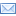SuperTeacherTools### 7th Grade Math Jeopardy Speed Match Review Game

This game has been played times
The best time ever was at seconds.
The best time today is at seconds.
How To Play:
Drag the squares in the gray area and drop them in the matching squares in the blue area below.
If you drop a square in the correct spot, it will disappear.
If you make a mistake, the square will return to its original spot.
Good luck!

Let's Play Speed Match!

378
Jonathan and Jameson
3
Mrs. Herrerra
\$55,250
Slope describes how much you go up and down, to how much you go over between two points.
200%
Mr. Olea
15/49
35/100
1/12
60
525,600 Minutes
Anaheim
-1/10
5
3/4
-3
2%
.10
8760 hours
Sacramento
4/9
Graph the points (4, -2) and (-5, -7)
What is our Principal's name?
18.9 / .05
How many hours are in a year?
How do you find slope?
A car is worth 65,000 dollars. If I get a teacher discount of 15%, how much do I pay for the car?
5/7 / 2 1/3 =
Write 10% as a decimal.
5k=25 What is k?
Two angles of a triangle are 58 and 62 what is the measurement of the last angle?
What fraction is greater 3/4 or 4/6?
7/9 + (-1/3) =
What are Ms. Jackson's boys names?
What is the capital of California?
3 + 7 + 9 + 3 - 12 + 3 - 10 =
Find the slope of (-6,3) and (-3, -6)
Write 35% as a fraction.
Does this work? (-4,9) 3x + 4y = 24
Write .02 as a precentage.
50 is what percent of 25?
Find the slope of (-6, 3) and (4,2)
Who teaches in the room underneath Ms. Jackson's room?
1/4 - 1/6 =
What city in California is Disneyland located?
How many minutes are in a year?

## You did it!What is your name?

## Here are all the answers:

 Description Match: Write 10% as a decimal. .10 Write 35% as a fraction. 35/100 Write .02 as a precentage. 2% 50 is what percent of 25? 200% A car is worth 65,000 dollars. If I get a teacher discount of 15%, how much do I pay for the car? \$55,250 What fraction is greater 3/4 or 4/6? 3/4 1/4 - 1/6 = 1/12 7/9 + (-1/3) = 4/9 5/7 / 2 1/3 = 15/49 18.9 / .05 378 Find the slope of (-6, 3) and (4,2) -1/10 How do you find slope? Slope describes how much you go up and down, to how much you go over between two points. Find the slope of (-6,3) and (-3, -6) -3 3 + 7 + 9 + 3 - 12 + 3 - 10 = 3 How many hours are in a year? 8760 hours Two angles of a triangle are 58 and 62 what is the measurement of the last angle? 60 5k=25 What is k? 5 How many minutes are in a year? 525,600 Minutes What is our Principal's name? Mrs. Herrerra What is the capital of California? Sacramento What are Ms. Jackson's boys names? Jonathan and Jameson Who teaches in the room underneath Ms. Jackson's room? Mr. Olea What city in California is Disneyland located? Anaheim